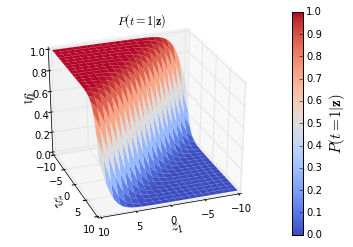Tags: , ,

# How to implement a neural network Intermezzo 2

This page is part of a 5 (+2) parts tutorial on how to implement a simple neural network model. You can find the links to the rest of the tutorial here:

## Softmax classification function

This intermezzo will cover:

The previous intermezzo described how to do a classification of 2 classes with the help of the logistic function . For multiclass classification there exists an extension of this logistic function called the softmax function which is used in multinomial logistic regression . The following section will explain the softmax function and how to derive it.

In :

## Softmax function

The logistic output function described in the previous intermezzo can only be used for the classification between two target classes t=1

and t=0. This logistic function can be generalized to output a multiclass categorical probability distribution by the softmax function . This softmax function ς takes as input a C-dimensional vector z and outputs a C-dimensional vector y of real values between 0 and 1

. This function is a normalized exponential and is defined as:

yc=ς(z)c=ezcCd=1ezdforc=1C

The denominator Cd=1ezd

acts as a regularizer to make sure that Cc=1yc=1. As the output layer of a neural network, the softmax function can be represented graphically as a layer with C

neurons.

We can write the probabilities that the class is t=c

for c=1C given input z

as:

⎡⎣⎢⎢⎢P(t=1|z)P(t=C|z)⎤⎦⎥⎥⎥=⎡⎣⎢⎢⎢ς(z)1ς(z)C⎤⎦⎥⎥⎥=1Cd=1ezd⎡⎣⎢⎢⎢ez1ezC⎤⎦⎥⎥⎥

Where P(t=c|z)

is thus the probability that that the class is c given the input z

.

These probabilities of the output P(t=1|z)

for an example system with 2 classes (t=1, t=2) and input z=[z1,z2] is shown in the figure below. The other probability P(t=2|z)

will be complementary.

In :
In :### Derivative of the softmax function

To use the softmax function in neural networks, we need to compute its derivative. If we define ΣC=Cd=1ezdforc=1C

so that yc=ezc/ΣC, then this derivative yi/zj of the output y of the softmax function with respect to its input z

can be calculated as:

ifi=j:ifij:yizi=eziΣCzi=eziΣCezieziΣ2C=eziΣCΣCeziΣC=eziΣC(1eziΣC)=yi(1yi)yizj=eziΣCzj=0eziezjΣ2C=eziΣCezjΣC=yiyj

Note that if i=j

this derivative is similar to the derivative of the logistic function.

### Cross-entropy cost function for the softmax function

To derive the cost function for the softmax function we start out from the likelihood function that a given set of parameters θ

of the model can result in prediction of the correct class of each input sample, as in the derivation for the logistic cost function. The maximization of this likelihood can be written as:

argmaxθ(θ|t,z)

The likelihood (θ|t,z)

can be rewritten as the joint probability of generating t and z given the parameters θ: P(t,z|θ)

. Which can be written as a conditional distribution:

P(t,z|θ)=P(t|z,θ)P(z|θ)

Since we are not interested in the probability of z

we can reduce this to: (θ|t,z)=P(t|z,θ). Which can be written as P(t|z) for fixed θ. Since each ti is dependent on the full z, and only 1 class can be activated in the t

we can write

P(t|z)=i=cCP(tc|z)tc=i=cCς(z)tcc=i=cCytcc

As was noted during the derivation of the cost function of the logistic function, maximizing this likelihood can also be done by minimizing the negative log-likelihood:

log(θ|t,z)=ξ(t,z)=logi=cCytcc=i=cCtclog(yc)

Which is the cross-entropy error function ξ

. Note that for a 2 class system output t2=1t1 and this results in the same error function as for logistic regression: ξ(t,y)=tclog(yc)(1tc)log(1yc)

.

The cross-entropy error function over a batch of multiple samples of size n

can be calculated as:

ξ(T,Y)=i=1nξ(ti,yi)=i=1ni=cCticlog(yic)

Where tic

is 1 if and only if sample i belongs to class c, and yic is the output probability that sample i belongs to class c

.

#### Derivative of the cross-entropy cost function for the softmax function

The derivative ξ/zi

of the cost function with respect to the softmax input zi

can be calculated as:

ξzi=j=1Ctjlog(yj)zi=j=1Ctjlog(yj)zi=j=1Ctj1yjyjzi=tiyiyizijiCtjyjyjzi=tiyiyi(1yi)jiCtjyj(yjyi)=ti+tiyi+jiCtjyi=ti+j=1Ctjyi=ti+yij=1Ctj=yiti

Note that we already derived yj/zi

for i=j and ij

above.

The result that ξ/zi=yiti

for all iC

is the same as the derivative of the cross-entropy for the logistic function which had only one output node.

This post at peterroelants.github.io is generated from an IPython notebook file. Link to the full IPython notebook file